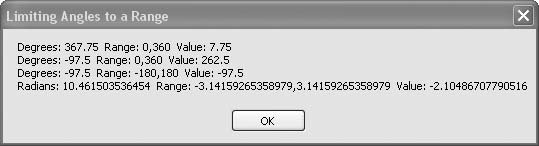# Recipe 6.11. Limiting Angles to a Range

#### Problem

You want to shift intermediate angular calculation results into a range such as 0° to 360°, -180° to 180°, 0 to 2π radians, or π to π radians.

#### Solution

Sample code folder: Chapter 06\AngleRange

Create a function that handles all these range conversions efficiently.

#### Discussion

Some scientific calculations produce angular results that are beyond normal ranges, requiring adjustment to bring them into the standard range of values. For example, in astronomical calculations a variety of polynomials are used to compute highly accurate positions of the planets and stars, but the polynomials often return angles representing many revolutions of the various orbs. You might say the angles are astronomical in size before they are adjusted into a normalized range such as 0° to 360°. The following function handles these range adjustments efficiently, bringing the values back down to earth:

` Public Function FixRange(ByVal origValue As Double, _       ByVal rangeMin As Double, ByVal rangeMax As Double) _       As Double    ' ----- Adjust a value to within a specified range.    '       Use the range size as the adjustment factor.    Dim shiftedValue As Double    Dim delta As Double    shiftedValue = origValue - rangeMin    delta = rangeMax - rangeMin    Return (((shiftedValue   Mod delta) + delta) Mod delta) + _       rangeMin End Function `

The FixRange() function accepts an out-of-range angular value expressed in either degrees or radians (or any range-limited system), followed by the minimum and maximum limits of the desired normalized range. All three parameters must use the same measurement system, such as radians, for the results to make sense.

The function uses a double application of the Mod operator plus some additions and subtractions to bring the value into the desired range. This calculation is more straightforward and efficient than adding or subtracting values in a loop until the value is brought into range, which is the technique sometimes shown in astronomical calculation books.

The following code demonstrates the use of the Range() function on a variety of positive and negative angular values as they are brought into a number of desired ranges:

` Dim result As New System.Text.StringBuilder Dim formatDegrees As String = _    "Degrees: {0} Range: {1},{2} Value: {3}" Dim formatRadians As String = _    "Radians: {0} Range: {1},{2} Value: {3}" Dim degrees As Double Dim radians As Double Dim ranged As Double ' ----- Degrees over the range. degrees = 367.75 ranged = FixRange(degrees, 0, 360) result.AppendLine(String.Format(formatDegrees, _    degrees, 0, 360, ranged)) ' ----- Degress under the range. degrees = -97.5 ranged = FixRange(degrees, 0, 360) result.AppendLine(String.Format(formatDegrees, _    degrees, 0, 360, ranged)) ' ----- Degrees in range. degrees = -97.5 ranged = FixRange(degrees, -180, 180) result.AppendLine(String.Format(formatDegrees, _    degrees, -180, 180, ranged)) ' ----- Radians over the range. radians = Math.PI * 3.33 ranged = FixRange(radians, -Math.PI, Math.PI) result.AppendLine(String.Format(formatRadians, _    radians, -Math.PI, Math.PI, ranged)) MsgBox(result.ToString()) `

Figure 6-11 shows the results produced by this sample code.

##### Figure 6-11. Using the Range( ) function to normalize angles in degrees or radiansSearch for information on the Mod operator in Visual Studio Help.Visual Basic 2005 Cookbook: Solutions for VB 2005 Programmers (Cookbooks (OReilly))
ISBN: 0596101775
EAN: 2147483647
Year: 2006
Pages: 400

Similar book on Amazon